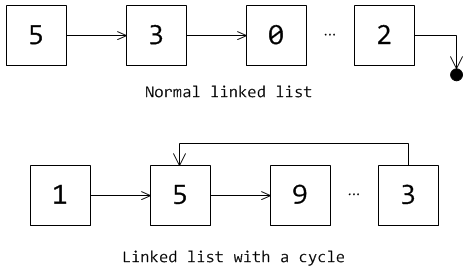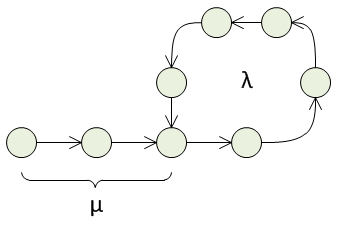# Finding a cycle in a linked list

Page content

There is a popular task at software developer job interviews: having a singly linked list, write a piece of code which tells if the list has a cycle.

In a linked list, each element is a structure which contains the value of an element and the link to the next element. The next-link of the last element has a special value which marks the end (usually, null). If a list has a cycle, the last element points to some element inside the list.How could this problem be solved? First of all, we could add visited flag to each element and traverse the list. This could solve the problem but requires modification of the elements which is usually unacceptable.

Secondly, we could use some external data structure like set to store the visited elements. This solution might work if we can identify elements uniquely and constantly. (Note: the value of an element generally cannot be its unique id). However, this approach has a drawback: we need O(N) space to store visited elements.

## The tortoise and the hare

Fortunately, it is possible to solve the problem without using additional storage. The algorithm known as “the tortoise and the hare” algorithm was proposed by Robert Floyd in 1967.

Let us consider a linked list with a cycle as having a tail μ items long and a cycle λ items long.If we put the pointer to the beginning of the list and start move it forward, it will come to the end of the tail and then will be going along the cycle endlessly.

It is obvious that there is a natural number m such that i = mλ ≥ μ. In other words, if we make i = mλ steps forward from the beginning we will pass (or at least reach) μ. At the point i, we can be sure that 2i is the same position on the cycle (due to integer number of full cycles: mλ and 2mλ). Let us take two pointers tortoise and hare and move the first one list element every step and the second twice as fast. If the list has a cycle, sooner or later (when they all are on the cycle and the distance between them is a multiple of λ) they will meet somewhere on it. This follows from the idea of i = mλ. So, the algorithm is quite simple.

def detect_cycle(head):

while hare:
tortoise = tortoise.next
hare = hare.next
if hare:
hare = hare.next
if tortoise is hare:
return True
return False

This algorithm needs only O(1) space and has O(μ + λ) time complexity.

## Exploring the cycle

What if we do not only want to know the fact if there is a cycle, but want to know values μ and λ.

Let us assume that there is a cycle in the list and do some calculations. We know that if we do i and 2i steps from the beginning we will be at the same point on the cycle. The way from the beginning to i consists of:

1. the tail μ;
2. p full circles λ, p ∈ N0 (natural numbers and zero);
3. k elements in the last piece of cycle, k ∈ N0.

What about 2i? It is the same point on the cycle, so μ and k parts are the same, the only difference is the number of full circles (let it be q instead of p).

i = μ + pλ + k,
2i = μ + qλ + k.

From this: 2(μ + pλ + k) = μ + qλ + k ⇔ 2μ + 2pλ + 2k = μ + qλ + k ⇔ μ = (q-2p)λ - k.

k is the part of the cycle at the beginning of it. Let r be the opposite part. So k = λ - r. μ = (q-2p)λ - λ + r = (q-2p-1)λ + r. We see that if we do some integer number of cycles (q-2p-1) from the meeting point of the tortoise and the hare and then r step to finish the cycle, we will do μ steps.

This could be used to find the length of the tail μ. Left the tortoise in the meeting point and move the hare back to the beginning of the list. Move them with the same speed (the hare is tired now) and they will meet at the beginning of the cycle.

A modified version of the code:

def detect_cycle_and_start(head):
# The function returns a tuple (Flag of cycle presence, mu value or None).

# Determine if there is a cycle.
while hare:
tortoise = tortoise.next
hare = hare.next
if hare:
hare = hare.next
if tortoise is hare:
print(tortoise)
break
else:
return (False, None)

# Determine the length of the tail mu.
mu = 0
while hare is not tortoise:
hare = hare.next
tortoise = tortoise.next
mu += 1
return (True, mu)

It is also possible to find λ, it is very simple: just place pointers at a random point on the cycle, fix one and move it, counting steps, another until they meet again.

## Applications

The problem is not only theoretical, there are some practical applications. For example, the algorithm could be used for detecting infinite loops in computer programs.

We can consider a sequence of some function applications instead of a list: f(f(...f(x))). The same algorithm can be used to find a cycle in it. This might help if you want to measure the strength of pseudorandom number generator and in other engineering and number-theoretic applications.